# 6.5 BiCS Memory Hole Etching

The etching of the BiCS memory hole is a combination of the SiO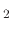and Si etching processes presented in Section 5.2. The initial structure which is to be etched is shown in Figure 6.20a. The width of the mask opening is 58nm, while the heights of the interchanging silicon and silicon dioxide layers are 50nm and 33nm, respectively. The silicon dioxide layer is etched using the model described in Section 5.2.1 and the silicon layer is etched with the model from Section 5.2.2 with the parameters listed in  and , respectively. Figure 6.20b shows the final topography after the sequence of processing steps are applied. The fluxes used for silicon etching are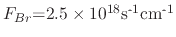,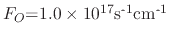, and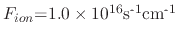. The fluxes used in the simulation of silicon dioxide etching are are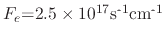,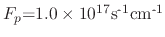, and. The silicon and silicon dioxide were etched for 60s and 6s, respectively.

Figure 6.20: The initial and final topographies after applying Si and SiOetching models for the fabrication of BiCS memory holes.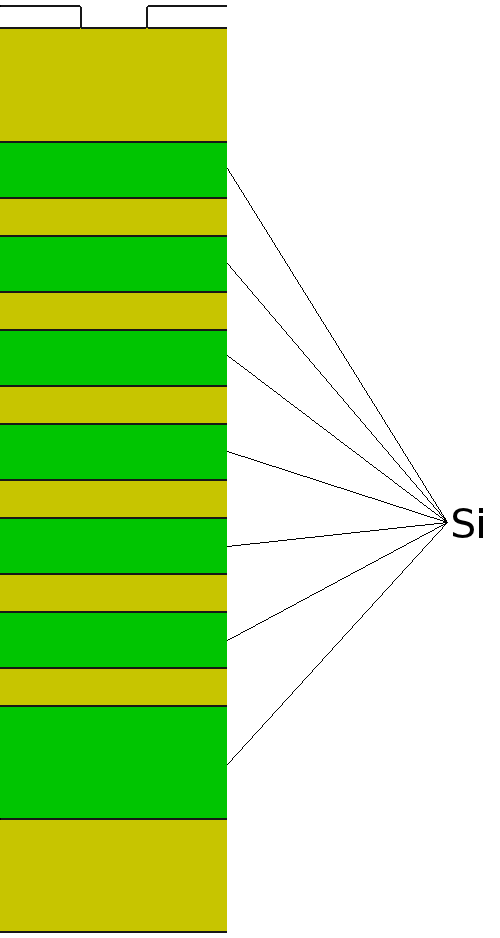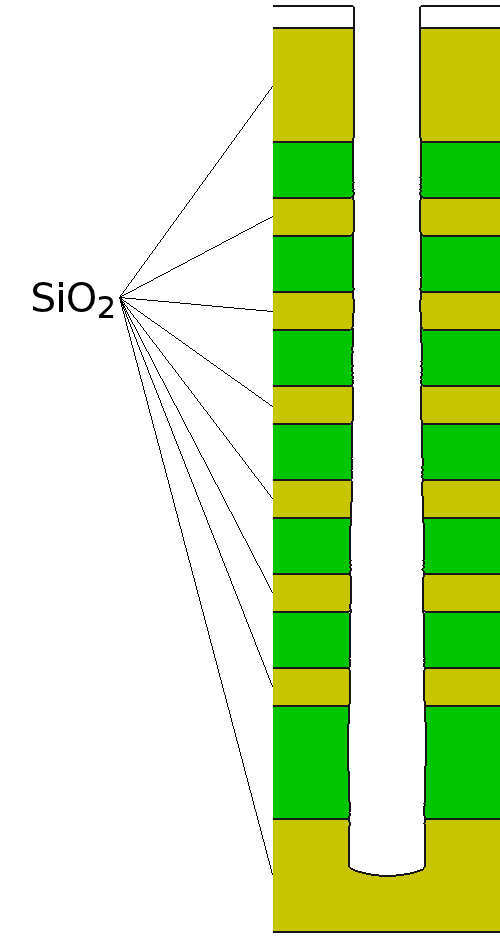(a) Initial topography (b) Topography after etching

L. Filipovic: Topography Simulation of Novel Processing Techniques